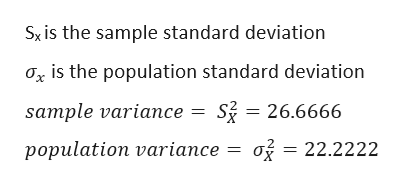# Use software or a calculator to find the​ range, variance, and standard deviation of the following body​temperatures, in degrees​ Fahrenheit, taken at​ 12:00 A.M.I don't remember how to find variance on a TI-83 Plus calculator and translate standard deviation. HELP!

Question
614 views

Use software or a calculator to find the​ range, variance, and standard deviation of the following body​temperatures, in degrees​ Fahrenheit, taken at​ 12:00 A.M.

I don't remember how to find variance on a TI-83 Plus calculator and translate standard deviation. HELP!

check_circle

Step 1

As data of temperature is not provided here. Here i can show you how to find the range, standard deviation and variance on Ti-83 calculator.

I have taken the following data for your reference

Step 2
Range is the difference of maximum value and minimum value from the data set.
Here Range = 39-25 = 14
Steps to perform descriptive statistics on T1-84 CALCULATOR
1. On Ti-84 calculator, press stat button and then enter.
2. Enter the data in L1 column and then press enter.
3. Again press on STAT button-then CALC and enter.
4. Click on 1 variable statistics
5. Then press on 2ND  button on calculator, choose L1 and then press enter,
6. Then the following value will be displayed on screen

Step 3

now calculate the va...help_outlineImage TranscriptioncloseSx is the sample standard deviation Ox is the population standard deviation Si 26.6666 sample variance o 22.2222 population variance fullscreen

### Want to see the full answer?

See Solution

#### Want to see this answer and more?

Solutions are written by subject experts who are available 24/7. Questions are typically answered within 1 hour.*

See Solution
*Response times may vary by subject and question.
Tagged in

### Data